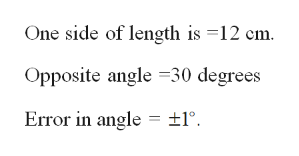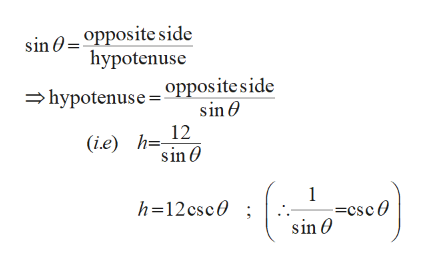# One side of a right triangle is known to be 12 cm long and the opposite angle is measured as 30°, with a possible error of ±1°.(a) Use differentials to estimate the error in computing the length of the hypotenuse. (Round your answer to two decimal places.)(b) What is the percentage error? (Round your answer to the nearest integer.)

Question
5 views

One side of a right triangle is known to be 12 cm long and the opposite angle is measured as 30°, with a possible error of ±1°.

(a) Use differentials to estimate the error in computing the length of the hypotenuse. (Round your answer to two decimal places.)

(b) What is the percentage error? (Round your answer to the nearest integer.)
check_circle

Step 1

Part (a):

To estimate the error in the length of hypotenuse.help_outlineImage TranscriptioncloseOne side of length is =12 cm. Opposite angle 30 degrees Error in angle ±1° fullscreen
Step 2

From the information,help_outlineImage Transcriptionclosesin 0=°Pposite side hypotenuse hypotenuse °ppositeside sin 12 (i.e) h=- sin 1 =csc0 sin h=12csc0 fullscreen
Step 3

Differentiate h with resp...

### Want to see the full answer?

See Solution

#### Want to see this answer and more?

Solutions are written by subject experts who are available 24/7. Questions are typically answered within 1 hour.*

See Solution
*Response times may vary by subject and question.
Tagged in

### Other